Anúncio

# Math 3 Curriculum Guide rev.2016

14 de Feb de 2019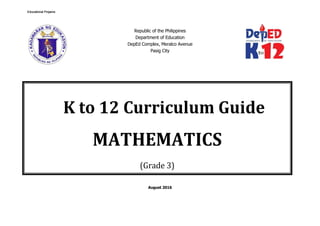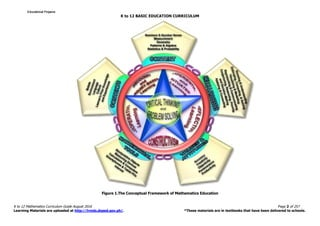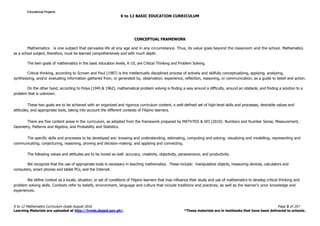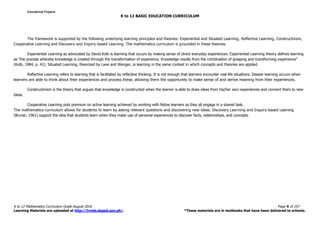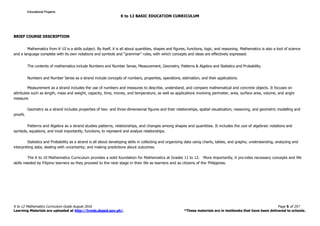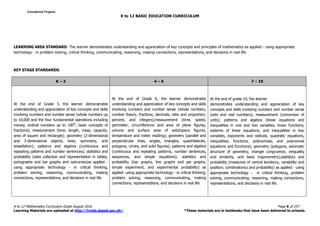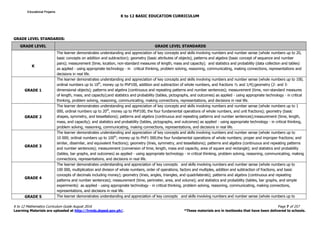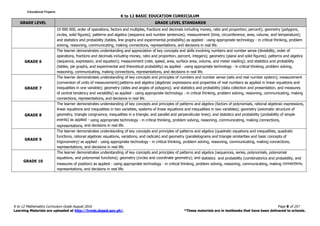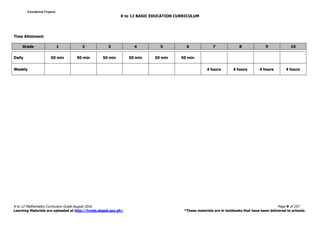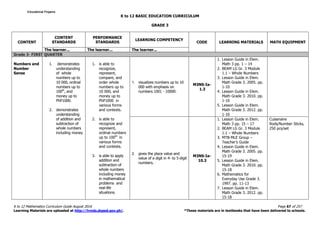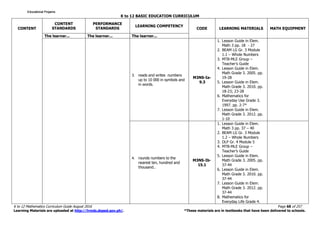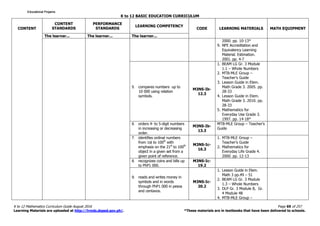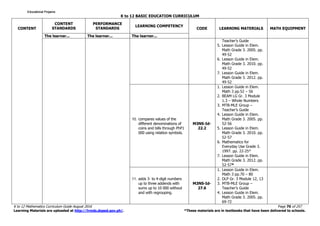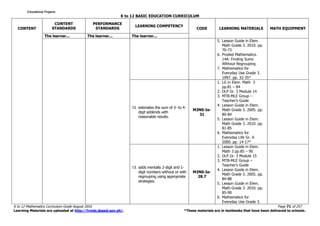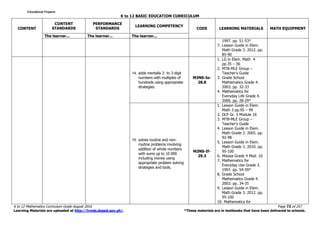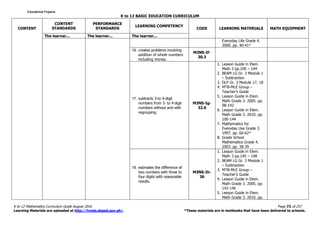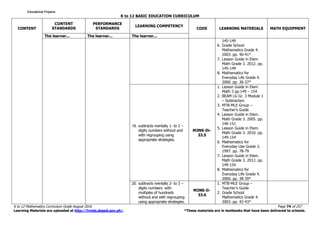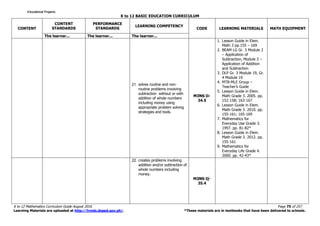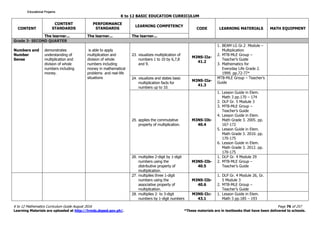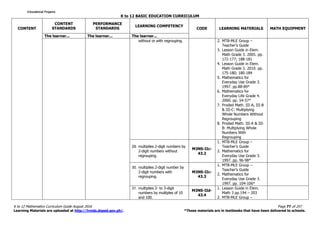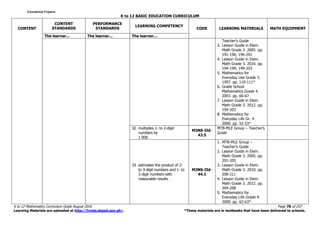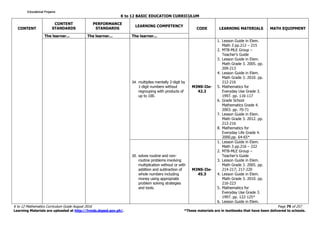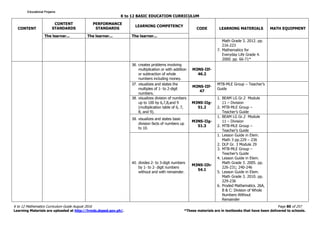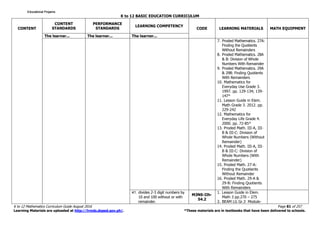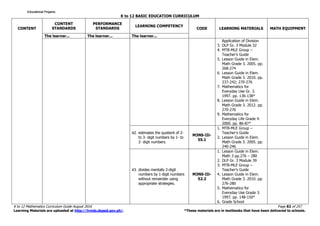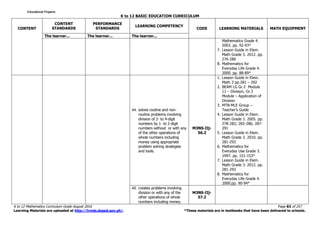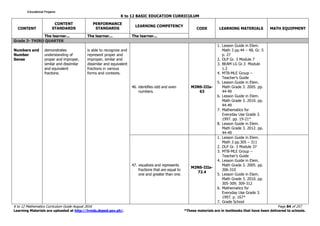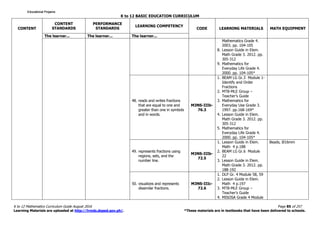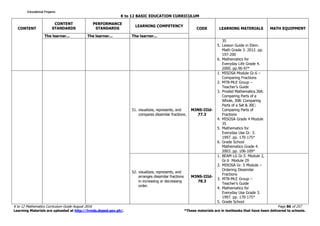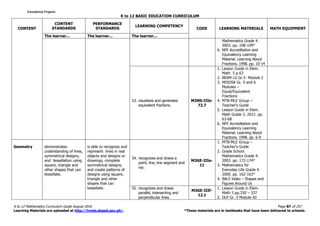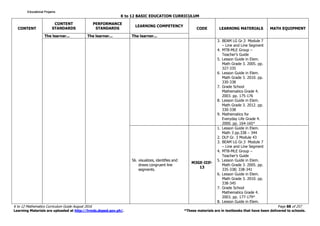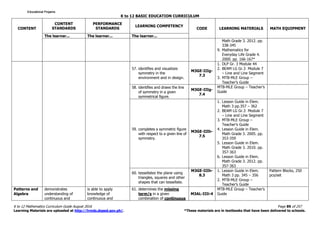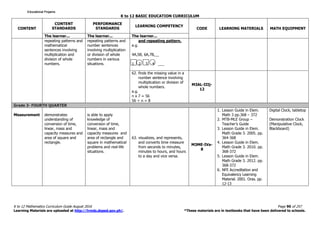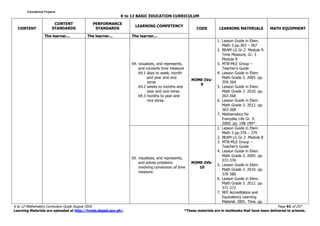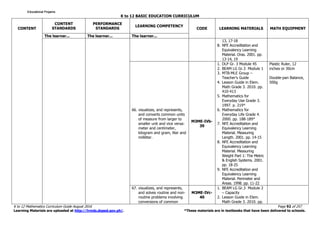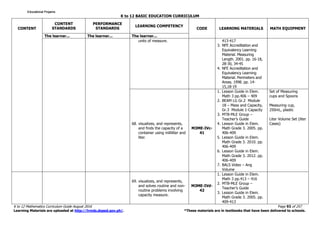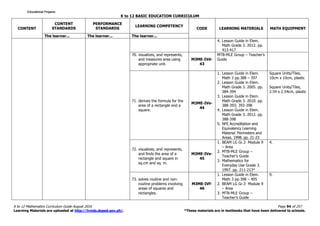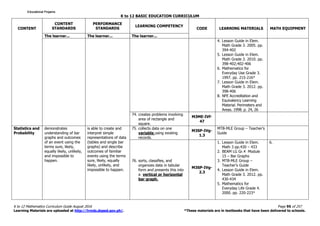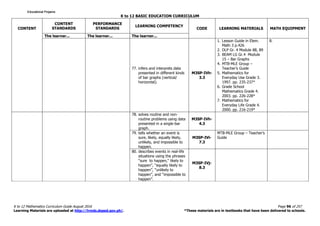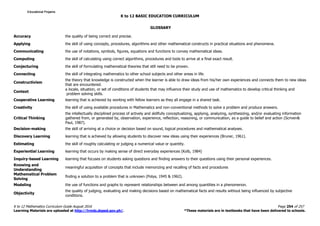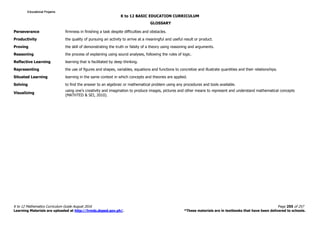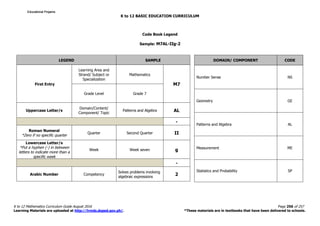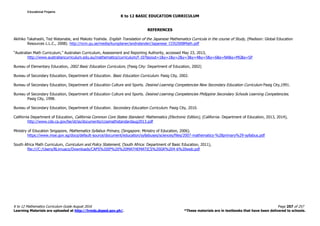1 de 43
Anúncio

### Math 3 Curriculum Guide rev.2016

1. Republic of the Philippines Department of Education DepEd Complex, Meralco Avenue Pasig City August 2016 K to 12 Curriculum Guide MATHEMATICS (Grade 3) Educational ProjamsEducational ProjamsEducational ProjamsEducational Projams
2. K to 12 BASIC EDUCATION CURRICULUM K to 12 Mathematics Curriculum Guide August 2016 Page 2 of 257 Learning Materials are uploaded at http://lrmds.deped.gov.ph/. *These materials are in textbooks that have been delivered to schools. Figure 1.The Conceptual Framework of Mathematics Education Educational ProjamsEducational ProjamsEducational ProjamsEducational Projams
3. K to 12 BASIC EDUCATION CURRICULUM K to 12 Mathematics Curriculum Guide August 2016 Page 3 of 257 Learning Materials are uploaded at http://lrmds.deped.gov.ph/. *These materials are in textbooks that have been delivered to schools. CONCEPTUAL FRAMEWORK Mathematics is one subject that pervades life at any age and in any circumstance. Thus, its value goes beyond the classroom and the school. Mathematics as a school subject, therefore, must be learned comprehensively and with much depth. The twin goals of mathematics in the basic education levels, K-10, are Critical Thinking and Problem Solving. Critical thinking, according to Scriven and Paul (1987) is the intellectually disciplined process of actively and skilfully conceptualizing, applying, analyzing, synthesizing, and/or evaluating information gathered from, or generated by, observation, experience, reflection, reasoning, or communication, as a guide to belief and action. On the other hand, according to Polya (1945 & 1962), mathematical problem solving is finding a way around a difficulty, around an obstacle, and finding a solution to a problem that is unknown. These two goals are to be achieved with an organized and rigorous curriculum content, a well-defined set of high-level skills and processes, desirable values and attitudes, and appropriate tools, taking into account the different contexts of Filipino learners. There are five content areas in the curriculum, as adopted from the framework prepared by MATHTED & SEI (2010): Numbers and Number Sense, Measurement, Geometry, Patterns and Algebra, and Probability and Statistics. The specific skills and processes to be developed are: knowing and understanding; estimating, computing and solving; visualizing and modelling; representing and communicating; conjecturing, reasoning, proving and decision-making; and applying and connecting. The following values and attitudes are to be honed as well: accuracy, creativity, objectivity, perseverance, and productivity. We recognize that the use of appropriate tools is necessary in teaching mathematics. These include: manipulative objects, measuring devices, calculators and computers, smart phones and tablet PCs, and the Internet. We define context as a locale, situation, or set of conditions of Filipino learners that may influence their study and use of mathematics to develop critical thinking and problem solving skills. Contexts refer to beliefs, environment, language and culture that include traditions and practices, as well as the learner’s prior knowledge and experiences. Educational ProjamsEducational ProjamsEducational ProjamsEducational Projams
4. K to 12 BASIC EDUCATION CURRICULUM K to 12 Mathematics Curriculum Guide August 2016 Page 4 of 257 Learning Materials are uploaded at http://lrmds.deped.gov.ph/. *These materials are in textbooks that have been delivered to schools. The framework is supported by the following underlying learning principles and theories: Experiential and Situated Learning, Reflective Learning, Constructivism, Cooperative Learning and Discovery and Inquiry-based Learning. The mathematics curriculum is grounded in these theories. Experiential Learning as advocated by David Kolb is learning that occurs by making sense of direct everyday experiences. Experiential Learning theory defines learning as "the process whereby knowledge is created through the transformation of experience. Knowledge results from the combination of grasping and transforming experience" (Kolb, 1984, p. 41). Situated Learning, theorized by Lave and Wenger, is learning in the same context in which concepts and theories are applied. Reflective Learning refers to learning that is facilitated by reflective thinking. It is not enough that learners encounter real-life situations. Deeper learning occurs when learners are able to think about their experiences and process these, allowing them the opportunity to make sense of and derive meaning from their experiences. Constructivism is the theory that argues that knowledge is constructed when the learner is able to draw ideas from his/her own experiences and connect them to new ideas. Cooperative Learning puts premium on active learning achieved by working with fellow learners as they all engage in a shared task. The mathematics curriculum allows for students to learn by asking relevant questions and discovering new ideas. Discovery Learning and Inquiry-based Learning (Bruner, 1961) support the idea that students learn when they make use of personal experiences to discover facts, relationships, and concepts. Educational ProjamsEducational ProjamsEducational ProjamsEducational Projams
5. K to 12 BASIC EDUCATION CURRICULUM K to 12 Mathematics Curriculum Guide August 2016 Page 5 of 257 Learning Materials are uploaded at http://lrmds.deped.gov.ph/. *These materials are in textbooks that have been delivered to schools. BRIEF COURSE DESCRIPTION Mathematics from K-10 is a skills subject. By itself, it is all about quantities, shapes and figures, functions, logic, and reasoning. Mathematics is also a tool of science and a language complete with its own notations and symbols and “grammar” rules, with which concepts and ideas are effectively expressed. The contents of mathematics include Numbers and Number Sense, Measurement, Geometry, Patterns & Algebra and Statistics and Probability. Numbers and Number Sense as a strand include concepts of numbers, properties, operations, estimation, and their applications. Measurement as a strand includes the use of numbers and measures to describe, understand, and compare mathematical and concrete objects. It focuses on attributes such as length, mass and weight, capacity, time, money, and temperature, as well as applications involving perimeter, area, surface area, volume, and angle measure. Geometry as a strand includes properties of two- and three-dimensional figures and their relationships, spatial visualization, reasoning, and geometric modelling and proofs. Patterns and Algebra as a strand studies patterns, relationships, and changes among shapes and quantities. It includes the use of algebraic notations and symbols, equations, and most importantly, functions, to represent and analyze relationships. Statistics and Probability as a strand is all about developing skills in collecting and organizing data using charts, tables, and graphs; understanding, analyzing and interpreting data; dealing with uncertainty; and making predictions about outcomes. The K to 10 Mathematics Curriculum provides a solid foundation for Mathematics at Grades 11 to 12. More importantly, it pro vides necessary concepts and life skills needed by Filipino learners as they proceed to the next stage in their life as learners and as citizens of the Philippines. Educational ProjamsEducational ProjamsEducational ProjamsEducational Projams
6. K to 12 BASIC EDUCATION CURRICULUM K to 12 Mathematics Curriculum Guide August 2016 Page 6 of 257 Learning Materials are uploaded at http://lrmds.deped.gov.ph/. *These materials are in textbooks that have been delivered to schools. LEARNING AREA STANDARD: The learner demonstrates understanding and appreciation of key concepts and principles of mathematics as applied - using appropriate technology - in problem solving, critical thinking, communicating, reasoning, making connections, representations, and decisions in real life. KEY STAGE STANDARDS: K – 3 4 – 6 7 – 10 At the end of Grade 3, the learner demonstrates understanding and appreciation of key concepts and skills involving numbers and number sense (whole numbers up to 10,000 and the four fundamental operations including money, ordinal numbers up to 100th , basic concepts of fractions); measurement (time, length, mass, capacity, area of square and rectangle); geometry (2-dimensional and 3-dimensional objects, lines, symmetry, and tessellation); patterns and algebra (continuous and repeating patterns and number sentences); statistics and probability (data collection and representation in tables, pictographs and bar graphs and outcomes)as applied - using appropriate technology - in critical thinking, problem solving, reasoning, communicating, making connections, representations, and decisions in real life. At the end of Grade 6, the learner demonstrates understanding and appreciation of key concepts and skills involving numbers and number sense (whole numbers, number theory, fractions, decimals, ratio and proportion, percent, and integers);measurement (time, speed, perimeter, circumference and area of plane figures, volume and surface area of solid/space figures, temperature and meter reading); geometry (parallel and perpendicular lines, angles, triangles, quadrilaterals, polygons, circles, and solid figures); patterns and algebra (continuous and repeating patterns, number sentences, sequences, and simple equations); statistics and probability (bar graphs, line graphs and pie graphs, simple experiment, and experimental probability) as applied -using appropriate technology - in critical thinking, problem solving, reasoning, communicating, making connections, representations, and decisions in real life. At the end of grade 10, the learner demonstrates understanding and appreciation of key concepts and skills involving numbers and number sense (sets and real numbers); measurement (conversion of units); patterns and algebra (linear equations and inequalities in one and two variables, linear functions, systems of linear equations, and inequalities in two variables, exponents and radicals, quadratic equations, inequalities, functions, polynomials, and polynomial equations and functions); geometry (polygons, axiomatic structure of geometry, triangle congruence, inequality and similarity, and basic trigonometry);statistics and probability (measures of central tendency, variability and position; combinatorics and probability) as applied - using appropriate technology - in critical thinking, problem solving, communicating, reasoning, making connections, representations, and decisions in real life. Educational ProjamsEducational ProjamsEducational ProjamsEducational Projams
9. K to 12 BASIC EDUCATION CURRICULUM K to 12 Mathematics Curriculum Guide August 2016 Page 9 of 257 Learning Materials are uploaded at http://lrmds.deped.gov.ph/. *These materials are in textbooks that have been delivered to schools. Daily 50 min 50 min 50 min 50 min 50 min 50 min Weekly 4 hours 4 hours 4 hours 4 hours Time Allotment: Grade 1 2 3 4 5 6 7 8 9 10 Educational ProjamsEducational ProjamsEducational ProjamsEducational Projams
11. K to 12 BASIC EDUCATION CURRICULUM K to 12 Mathematics Curriculum Guide August 2016 Page 68 of 257 Learning Materials are uploaded at http://lrmds.deped.gov.ph/. *These materials are in textbooks that have been delivered to schools. CONTENT CONTENT STANDARDS PERFORMANCE STANDARDS LEARNING COMPETENCY CODE LEARNING MATERIALS MATH EQUIPMENT The learner... The learner... The learner... 3. reads and writes numbers up to 10 000 in symbols and in words. M3NS-Ia- 9.3 1. Lesson Guide in Elem. Math 3 pp. 18 - 27 2. BEAM LG Gr. 3 Module 1.1 – Whole Numbers 3. MTB-MLE Group – Teacher’s Guide 4. Lesson Guide in Elem. Math Grade 3. 2005. pp. 19-28 5. Lesson Guide in Elem. Math Grade 3. 2010. pp. 18-23; 23-28 6. Mathematics for Everyday Use Grade 3. 1997. pp. 2-7* 7. Lesson Guide in Elem. Math Grade 3. 2012. pp. 1-10 4. rounds numbers to the nearest ten, hundred and thousand.. M3NS-Ib- 15.1 1. Lesson Guide in Elem. Math 3 pp. 37 – 40 2. BEAM LG Gr. 3 Module 1.2 – Whole Numbers 3. DLP Gr. 4 Module 5 4. MTB-MLE Group – Teacher’s Guide 5. Lesson Guide in Elem. Math Grade 3. 2005. pp. 37-44 6. Lesson Guide in Elem. Math Grade 3. 2010. pp. 37-44 7. Lesson Guide in Elem. Math Grade 3. 2012. pp. 37-44 8. Mathematics for Everyday Life Grade 4. Educational ProjamsEducational ProjamsEducational ProjamsEducational Projams
12. K to 12 BASIC EDUCATION CURRICULUM K to 12 Mathematics Curriculum Guide August 2016 Page 69 of 257 Learning Materials are uploaded at http://lrmds.deped.gov.ph/. *These materials are in textbooks that have been delivered to schools. CONTENT CONTENT STANDARDS PERFORMANCE STANDARDS LEARNING COMPETENCY CODE LEARNING MATERIALS MATH EQUIPMENT The learner... The learner... The learner... 2000. pp. 10-13* 9. NFE Accreditation and Equivalency Learning Material. Estimation. 2001. pp. 4-7 5. compares numbers up to 10 000 using relation symbols. M3NS-Ib- 12.3 1. BEAM LG Gr. 3 Module 1.1 – Whole Numbers 2. MTB-MLE Group – Teacher’s Guide 3. Lesson Guide in Elem. Math Grade 3. 2005. pp. 28-33 4. Lesson Guide in Elem. Math Grade 3. 2010. pp. 28-33 5. Mathematics for Everyday Use Grade 3. 1997. pp. 14-18* 6. orders 4- to 5-digit numbers in increasing or decreasing order. M3NS-Ib- 13.3 MTB-MLE Group – Teacher’s Guide 7. identifies ordinal numbers from 1st to 100th with emphasis on the 21st to 100th object in a given set from a given point of reference. M3NS-Ic- 16.3 1. MTB-MLE Group – Teacher’s Guide 2. Mathematics for Everyday Life Grade 4. 2000. pp. 12-13 8. recognizes coins and bills up to PhP1 000. M3NS-Ic- 19.2 9. reads and writes money in symbols and in words through PhP1 000 in pesos and centavos. M3NS-Ic- 20.2 1. Lesson Guide in Elem. Math 3 pp.49 – 51 2. BEAM LG Gr. 3 Module 1.3 – Whole Numbers 3. DLP Gr. 3 Module 8, Gr. 4 Module 48 4. MTB-MLE Group – Educational ProjamsEducational ProjamsEducational ProjamsEducational Projams
14. K to 12 BASIC EDUCATION CURRICULUM K to 12 Mathematics Curriculum Guide August 2016 Page 71 of 257 Learning Materials are uploaded at http://lrmds.deped.gov.ph/. *These materials are in textbooks that have been delivered to schools. CONTENT CONTENT STANDARDS PERFORMANCE STANDARDS LEARNING COMPETENCY CODE LEARNING MATERIALS MATH EQUIPMENT The learner... The learner... The learner... 5. Lesson Guide in Elem. Math Grade 3. 2010. pp. 70-73 6. Proded Mathematics. 14A: Finding Sums Without Regrouping 7. Mathematics for Everyday Use Grade 3. 1997. pp. 32-35* 12. estimates the sum of 3- to 4- digit addends with reasonable results. M3NS-Ie- 31 1. LG in Elem. Math 3 pp.81 – 84 2. DLP Gr. 3 Module 14 3. MTB-MLE Group – Teacher’s Guide 4. Lesson Guide in Elem. Math Grade 3. 2005. pp. 80-84 5. Lesson Guide in Elem. Math Grade 3. 2010. pp. 81-85 6. Mathematics for Everyday Life Gr. 4. 2000. pp. 14-17* 13. adds mentally 2-digit and 1- digit numbers without or with regrouping using appropriate strategies. M3NS-Ie- 28.7 1. Lesson Guide in Elem. Math 3 pp.85 – 90 2. DLP Gr. 3 Module 15 3. MTB-MLE Group – Teacher’s Guide 4. Lesson Guide in Elem. Math Grade 3. 2005. pp. 84-88 5. Lesson Guide in Elem. Math Grade 3. 2010. pp. 85-90 6. Mathematics for Everyday Use Grade 3. Educational ProjamsEducational ProjamsEducational ProjamsEducational Projams
16. K to 12 BASIC EDUCATION CURRICULUM K to 12 Mathematics Curriculum Guide August 2016 Page 73 of 257 Learning Materials are uploaded at http://lrmds.deped.gov.ph/. *These materials are in textbooks that have been delivered to schools. CONTENT CONTENT STANDARDS PERFORMANCE STANDARDS LEARNING COMPETENCY CODE LEARNING MATERIALS MATH EQUIPMENT The learner... The learner... The learner... Everyday Life Grade 4. 2000. pp. 40-41* 16. creates problems involving addition of whole numbers including money. M3NS-If- 30.3 17. subtracts 3-to 4-digit numbers from 3- to 4-digit numbers without and with regrouping. M3NS-Ig- 32.6 1. Lesson Guide in Elem. Math 3 pp.100 – 144 2. BEAM LG Gr. 3 Module 1 – Subtraction 3. DLP Gr. 3 Module 17, 18 4. MTB-MLE Group – Teacher’s Guide 5. Lesson Guide in Elem. Math Grade 3. 2005. pp. 98-142 6. Lesson Guide in Elem. Math Grade 3. 2010. pp. 100-144 7. Mathematics for Everyday Use Grade 3. 1997. pp. 60-62* 8. Grade School Mathematics Grade 4. 2003. pp. 38-39 18. estimates the difference of two numbers with three to four digits with reasonable results. M3NS-Ih- 36 1. Lesson Guide in Elem. Math 3 pp.145 – 148 2. BEAM LG Gr. 3 Module 1 – Subtraction 3. MTB-MLE Group – Teacher’s Guide 4. Lesson Guide in Elem. Math Grade 3. 2005. pp. 142-146 5. Lesson Guide in Elem. Math Grade 3. 2010. pp. Educational ProjamsEducational ProjamsEducational ProjamsEducational Projams
18. K to 12 BASIC EDUCATION CURRICULUM K to 12 Mathematics Curriculum Guide August 2016 Page 75 of 257 Learning Materials are uploaded at http://lrmds.deped.gov.ph/. *These materials are in textbooks that have been delivered to schools. CONTENT CONTENT STANDARDS PERFORMANCE STANDARDS LEARNING COMPETENCY CODE LEARNING MATERIALS MATH EQUIPMENT The learner... The learner... The learner... 21. solves routine and non- routine problems involving subtraction without or with addition of whole numbers including money using appropriate problem solving strategies and tools. M3NS-Ii- 34.5 1. Lesson Guide in Elem. Math 3 pp.155 – 169 2. BEAM LG Gr. 3 Module 2 – Application of Subtraction, Module 3 – Application of Addition and Subtraction 3. DLP Gr. 3 Module 19, Gr. 4 Module 19 4. MTB-MLE Group – Teacher’s Guide 5. Lesson Guide in Elem. Math Grade 3. 2005. pp. 152-158; 163-167 6. Lesson Guide in Elem. Math Grade 3. 2010. pp. 155-161; 165-169 7. Mathematics for Everyday Use Grade 3. 1997. pp. 81-82* 8. Lesson Guide in Elem. Math Grade 3. 2012. pp. 155-161 9. Mathematics for Everyday Life Grade 4. 2000. pp. 42-43* 22. creates problems involving addition and/or subtraction of whole numbers including money. M3NS-Ij- 35.4 Educational ProjamsEducational ProjamsEducational ProjamsEducational Projams
19. K to 12 BASIC EDUCATION CURRICULUM K to 12 Mathematics Curriculum Guide August 2016 Page 76 of 257 Learning Materials are uploaded at http://lrmds.deped.gov.ph/. *These materials are in textbooks that have been delivered to schools. CONTENT CONTENT STANDARDS PERFORMANCE STANDARDS LEARNING COMPETENCY CODE LEARNING MATERIALS MATH EQUIPMENT The learner... The learner... The learner... Grade 3- SECOND QUARTER Numbers and Number Sense demonstrates understanding of multiplication and division of whole numbers including money. is able to apply multiplication and division of whole numbers including money in mathematical problems and real-life situations 23. visualizes multiplication of numbers 1 to 10 by 6,7,8 and 9. M3NS-IIa- 41.2 1. BEAM LG Gr.2 Module – Multiplication 2. MTB-MLE Group – Teacher’s Guide 3. Mathematics for Everyday Life Grade 2. 1999. pp.72-77* 24. visualizes and states basic multiplication facts for numbers up to 10. M3NS-IIa- 41.3 MTB-MLE Group – Teacher’s Guide 25. applies the commutative property of multiplication. M3NS-IIb- 40.4 1. Lesson Guide in Elem. Math 3 pp.170 – 174 2. DLP Gr. 5 Module 3 3. MTB-MLE Group – Teacher’s Guide 4. Lesson Guide in Elem. Math Grade 3. 2005. pp. 167-172 5. Lesson Guide in Elem. Math Grade 3. 2010. pp. 170-175 6. Lesson Guide in Elem. Math Grade 3. 2012. pp. 170-175 26. multiplies 2-digit by 1-digit numbers using the distributive property of multiplication. M3NS-IIb- 40.5 1. DLP Gr. 4 Module 29 2. MTB-MLE Group – Teacher’s Guide 27. multiplies three 1-digit numbers using the associative property of multiplication. M3NS-IIb- 40.6 1. DLP Gr. 4 Module 26, Gr. 5 Module 3 2. MTB-MLE Group – Teacher’s Guide 28. multiplies 2- to 3-digit numbers by 1-digit numbers M3NS-IIc- 43.1 1. Lesson Guide in Elem. Math 3 pp.185 – 193 Educational ProjamsEducational ProjamsEducational ProjamsEducational Projams
20. K to 12 BASIC EDUCATION CURRICULUM K to 12 Mathematics Curriculum Guide August 2016 Page 77 of 257 Learning Materials are uploaded at http://lrmds.deped.gov.ph/. *These materials are in textbooks that have been delivered to schools. CONTENT CONTENT STANDARDS PERFORMANCE STANDARDS LEARNING COMPETENCY CODE LEARNING MATERIALS MATH EQUIPMENT The learner... The learner... The learner... without or with regrouping. 2. MTB-MLE Group – Teacher’s Guide 3. Lesson Guide in Elem. Math Grade 3. 2005. pp. 172-177; 188-181 4. Lesson Guide in Elem. Math Grade 3. 2010. pp. 175-180; 180-184 5. Mathematics for Everyday Use Grade 3. 1997. pp.88-89* 6. Mathematics for Everyday Life Grade 4. 2000. pp. 54-57* 7. Proded Math. III-A, III-B & III-C: Multiplying Whole Numbers Without Regrouping 8. Proded Math. III-A & III- B: Multiplying Whole Numbers With Regrouping 29. multiplies 2-digit numbers by 2-digit numbers without regrouping. M3NS-IIc- 43.2 1. MTB-MLE Group – Teacher’s Guide 2. Mathematics for Everyday Use Grade 3. 1997. pp. 96-98* 30. multiplies 2-digit number by 2-digit numbers with regrouping. M3NS-IIc- 43.3 1. MTB-MLE Group – Teacher’s Guide 2. Mathematics for Everyday Use Grade 3. 1997. pp. 104-106* 31. multiplies 2- to 3-digit numbers by multiples of 10 and 100. M3NS-IId- 43.4 1. Lesson Guide in Elem. Math 3 pp.194 – 203 2. MTB-MLE Group – Educational ProjamsEducational ProjamsEducational ProjamsEducational Projams
23. K to 12 BASIC EDUCATION CURRICULUM K to 12 Mathematics Curriculum Guide August 2016 Page 80 of 257 Learning Materials are uploaded at http://lrmds.deped.gov.ph/. *These materials are in textbooks that have been delivered to schools. CONTENT CONTENT STANDARDS PERFORMANCE STANDARDS LEARNING COMPETENCY CODE LEARNING MATERIALS MATH EQUIPMENT The learner... The learner... The learner... Math Grade 3. 2012. pp. 216-223 7. Mathematics for Everyday Life Grade 4. 2000. pp. 66-71* 36. creates problems involving multiplication or with addition or subtraction of whole numbers including money. M3NS-IIf- 46.2 37. visualizes and states the multiples of 1- to 2-digit numbers. M3NS-IIf- 47 MTB-MLE Group – Teacher’s Guide 38. visualizes division of numbers up to 100 by 6,7,8,and 9 (multiplication table of 6, 7, 8, and 9). M3NS-IIg- 51.2 1. BEAM LG Gr.2 Module 11 – Division 2. MTB-MLE Group – Teacher’s Guide 39. visualizes and states basic division facts of numbers up to 10. M3NS-IIg- 51.3 1. BEAM LG Gr.2 Module 11 – Division 2. MTB-MLE Group – Teacher’s Guide 40. divides 2- to 3-digit numbers by 1- to 2- digit numbers without and with remainder. M3NS-IIh- 54.1 1. Lesson Guide in Elem. Math 3 pp.229 – 236 2. DLP Gr. 3 Module 29 3. MTB-MLE Group – Teacher’s Guide 4. Lesson Guide in Elem. Math Grade 3. 2005. pp. 226-231; 240-246 5. Lesson Guide in Elem. Math Grade 3. 2010. pp. 229-236 6. Proded Mathematics. 26A, B & C: Division of Whole Numbers Without Remainder Educational ProjamsEducational ProjamsEducational ProjamsEducational Projams
24. K to 12 BASIC EDUCATION CURRICULUM K to 12 Mathematics Curriculum Guide August 2016 Page 81 of 257 Learning Materials are uploaded at http://lrmds.deped.gov.ph/. *These materials are in textbooks that have been delivered to schools. CONTENT CONTENT STANDARDS PERFORMANCE STANDARDS LEARNING COMPETENCY CODE LEARNING MATERIALS MATH EQUIPMENT The learner... The learner... The learner... 7. Proded Mathematics. 27A: Finding the Quotients Without Remainders 8. Proded Mathematics. 28A & B: Division of Whole Numbers With Remainder 9. Proded Mathematics. 29A & 29B: Finding Quotients With Remainders 10. Mathematics for Everyday Use Grade 3. 1997. pp. 129-134; 139- 147* 11. Lesson Guide in Elem. Math Grade 3. 2012. pp. 229-242 12. Mathematics for Everyday Life Grade 4. 2000. pp. 72-85* 13. Proded Math. III-A, III- B & III-C: Division of Whole Numbers (Without Remainder) 14. Proded Math. III-A, III- B & III-C: Division of Whole Numbers (With Remainder) 15. Proded Math. 27-A: Finding the Quotients Without Remainder 16. Proded Math. 29-A & 29-B: Finding Quotients With Remainders 41. divides 2-3 digit numbers by 10 and 100 without or with remainder. M3NS-IIh- 54.2 1. Lesson Guide in Elem. Math 3 pp.270 – 275 2. BEAM LG Gr.3 Module- Educational ProjamsEducational ProjamsEducational ProjamsEducational Projams
25. K to 12 BASIC EDUCATION CURRICULUM K to 12 Mathematics Curriculum Guide August 2016 Page 82 of 257 Learning Materials are uploaded at http://lrmds.deped.gov.ph/. *These materials are in textbooks that have been delivered to schools. CONTENT CONTENT STANDARDS PERFORMANCE STANDARDS LEARNING COMPETENCY CODE LEARNING MATERIALS MATH EQUIPMENT The learner... The learner... The learner... Application of Division 3. DLP Gr. 3 Module 32 4. MTB-MLE Group – Teacher’s Guide 5. Lesson Guide in Elem. Math Grade 3. 2005. pp. 268-274 6. Lesson Guide in Elem. Math Grade 3. 2010. pp. 237-242; 270-276 7. Mathematics for Everyday Use Gr. 3. 1997. pp. 136-138* 8. Lesson Guide in Elem. Math Grade 3. 2012. pp. 270-276 9. Mathematics for Everyday Life Grade 4. 2000. pp. 86-87* 42. estimates the quotient of 2- to 3- digit numbers by 1- to 2- digit numbers. M3NS-IIi- 55.1 1. MTB-MLE Group – Teacher’s Guide 2. Lesson Guide in Elem. Math Grade 3. 2005. pp. 240-246 43. divides mentally 2-digit numbers by 1-digit numbers without remainder using appropriate strategies. M3NS-IIi- 52.2 1. Lesson Guide in Elem. Math 3 pp.276 – 280 2. DLP Gr. 3 Module 39 3. MTB-MLE Group – Teacher’s Guide 4. Lesson Guide in Elem. Math Grade 3. 2010. pp. 276-280 5. Mathematics for Everyday Use Grade 3. 1997. pp. 148-150* 6. Grade School Educational ProjamsEducational ProjamsEducational ProjamsEducational Projams
26. K to 12 BASIC EDUCATION CURRICULUM K to 12 Mathematics Curriculum Guide August 2016 Page 83 of 257 Learning Materials are uploaded at http://lrmds.deped.gov.ph/. *These materials are in textbooks that have been delivered to schools. CONTENT CONTENT STANDARDS PERFORMANCE STANDARDS LEARNING COMPETENCY CODE LEARNING MATERIALS MATH EQUIPMENT The learner... The learner... The learner... Mathematics Grade 4. 2003. pp. 92-93* 7. Lesson Guide in Elem. Math Grade 3. 2012. pp. 276-280 8. Mathematics for Everyday Life Grade 4. 2000. pp. 88-89* 44. solves routine and non- routine problems involving division of 2- to 4-digit numbers by 1- to 2-digit numbers without or with any of the other operations of whole numbers including money using appropriate problem solving strategies and tools. M3NS-IIj- 56.2 1. Lesson Guide in Elem. Math 3 pp.281 – 292 2. BEAM LG Gr.2 Module 11 – Division, Gr.3 Module – Application of Division 3. MTB-MLE Group – Teacher’s Guide 4. Lesson Guide in Elem. Math Grade 3. 2005. pp. 278-282; 283-286; 287- 291 5. Lesson Guide in Elem. Math Grade 3. 2010. pp. 281-293 6. Mathematics for Everyday Use Grade 3. 1997. pp. 151-153* 7. Lesson Guide in Elem. Math Grade 3. 2012. pp. 281-293 8. Mathematics for Everyday Life Grade 4. 2000.pp. 90-94* 45. creates problems involving division or with any of the other operations of whole numbers including money. M3NS-IIj- 57.2 Educational ProjamsEducational ProjamsEducational ProjamsEducational Projams
27. K to 12 BASIC EDUCATION CURRICULUM K to 12 Mathematics Curriculum Guide August 2016 Page 84 of 257 Learning Materials are uploaded at http://lrmds.deped.gov.ph/. *These materials are in textbooks that have been delivered to schools. CONTENT CONTENT STANDARDS PERFORMANCE STANDARDS LEARNING COMPETENCY CODE LEARNING MATERIALS MATH EQUIPMENT The learner... The learner... The learner... Grade 3- THIRD QUARTER Numbers and Number Sense demonstrates understanding of proper and improper, similar and dissimilar and equivalent fractions. is able to recognize and represent proper and improper, similar and dissimilar and equivalent fractions in various forms and contexts. 46. identifies odd and even numbers. M3NS-IIIa- 63 1. Lesson Guide in Elem. Math 3 pp.44 – 48, Gr. 5 p. 27 2. DLP Gr. 3 Module 7 3. BEAM LG Gr.3 Module 1.2 4. MTB-MLE Group – Teacher’s Guide 5. Lesson Guide in Elem. Math Grade 3. 2005. pp. 44-49 6. Lesson Guide in Elem. Math Grade 3. 2010. pp. 44-49 7. Mathematics for Everyday Use Grade 3. 1997. pp. 19-21* 8. Lesson Guide in Elem. Math Grade 3. 2012. pp. 44-49 47. visualizes and represents fractions that are equal to one and greater than one. M3NS-IIIa- 72.4 1. Lesson Guide in Elem. Math 3 pp.305 – 311 2. DLP Gr. 3 Module 37 3. MTB-MLE Group – Teacher’s Guide 4. Lesson Guide in Elem. Math Grade 3. 2005. pp. 306-310 5. Lesson Guide in Elem. Math Grade 3. 2010. pp. 305-309; 309-312 6. Mathematics for Everyday Use Grade 3. 1997. p. 167* 7. Grade School Educational ProjamsEducational ProjamsEducational ProjamsEducational Projams
29. K to 12 BASIC EDUCATION CURRICULUM K to 12 Mathematics Curriculum Guide August 2016 Page 86 of 257 Learning Materials are uploaded at http://lrmds.deped.gov.ph/. *These materials are in textbooks that have been delivered to schools. CONTENT CONTENT STANDARDS PERFORMANCE STANDARDS LEARNING COMPETENCY CODE LEARNING MATERIALS MATH EQUIPMENT The learner... The learner... The learner... 35 5. Lesson Guide in Elem. Math Grade 3. 2012. pp. 197-200 6. Mathematics for Everyday Life Grade 4. 2000. pp.96-97* 51. visualizes, represents, and compares dissimilar fractions. M3NS-IIId- 77.3 1. MISOSA Module Gr.6 – Comparing Fractions 2. MTB-MLE Group – Teacher’s Guide 3. Proded Mathematics.30A: Comparing Parts of a Whole, 30B: Comparing Parts of a Set & 30C: Comparing Parts of Fractions 4. MISOSA Grade 4 Module 35 5. Mathematics for Everyday Use Gr. 3. 1997. pp. 170-175* 6. Grade School Mathematics Grade 4. 2003. pp. 106-109* 52. visualizes, represents, and arranges dissimilar fractions in increasing or decreasing order. M3NS-IIId- 78.3 1. BEAM LG Gr.5 Module 2, Gr.6 Module 29 2. MISOSA Gr. 5 Module – Ordering Dissimilar Fractions 3. MTB-MLE Group – Teacher’s Guide 4. Mathematics for Everyday Use Grade 3. 1997. pp. 170-175* 5. Grade School Educational ProjamsEducational ProjamsEducational ProjamsEducational Projams
30. K to 12 BASIC EDUCATION CURRICULUM K to 12 Mathematics Curriculum Guide August 2016 Page 87 of 257 Learning Materials are uploaded at http://lrmds.deped.gov.ph/. *These materials are in textbooks that have been delivered to schools. CONTENT CONTENT STANDARDS PERFORMANCE STANDARDS LEARNING COMPETENCY CODE LEARNING MATERIALS MATH EQUIPMENT The learner... The learner... The learner... Mathematics Grade 4. 2003. pp. 108-109* 6. NFE Accreditation and Equivalency Learning Material. Learning About Fractions. 1998. pp. 10-14 53. visualizes and generates equivalent fractions. M3NS-IIIe- 72.7 1. Lesson Guide in Elem. Math 5 p.63 2. BEAM LG Gr.5 Module 2 3. MISOSA Gr. 5 and 6 Modules – Equal/Equivalent Fractions 4. MTB-MLE Group – Teacher’s Guide 5. Lesson Guide in Elem. Math Grade 3. 2012. pp. 63-68 6. NFE Accreditation and Equivalency Learning Material. Learning About Fractions. 1998. pp. 6-9 Geometry demonstrates understanding of lines, symmetrical designs, and tessellation using square, triangle and other shapes that can tessellate. is able to recognize and represent lines in real objects and designs or drawings, complete symmetrical designs, and create patterns of designs using square, triangle and other shapes that can tessellate. 54. recognizes and draws a point, line, line segment and ray. M3GE-IIIe- 11 1. MTB-MLE Group – Teacher’s Guide 2. Grade School Mathematics Grade 4. 2003. pp. 172-174* 3. Mathematics for Everyday Life Grade 4. 2000. pp. 162-163* 4. BALS Video – Shapes and Figures Around Us 55. recognizes and draws parallel, intersecting and perpendicular lines. M3GE-IIIf- 12.1 1. Lesson Guide in Elem. Math 3 pp.330 – 337 2. DLP Gr. 3 Module 42 Educational ProjamsEducational ProjamsEducational ProjamsEducational Projams